Extent

概要

An extent is a rectangle specified by providing the coordinate of the lower left corner and the coordinate of the upper right corner in map units.

ディスカッション

メモ:

When used as input to ArcGIS Spatial Analyst エクステンション tools—Create Constant Raster, Create Normal Raster, Create Random Raster, Extract By Rectangle, and Topo To Raster—only the XMin, YMin, XMax, and YMax values are used by these tools.

構文

Extent  ({XMin}, {YMin}, {XMax}, {YMax}, {ZMin}, {ZMax}, {MMin}, {MMax}, {spatial_reference})
 パラメーター 説明 データ タイプ XMin The extent XMin value. Double YMin The extent YMin value. Double XMax The extent XMax value. Double YMax The extent YMax value. Double ZMin The extent ZMin value. None if no z-value. Double ZMax The extent ZMax value. None if no z-value. Double MMin The extent MMin value. None if no m-value. Double MMax The extent MMax value. None if no m-value. Double spatial_reference The spatial reference of the extent. SpatialReference

プロパティ

プロパティ説明データ タイプ
JSON
(読み取り専用)

Returns a JSON representation of the extent as a string.

ヒント:

The returned string can be converted to a dictionary using the Python json.loads function.

String
MMax
(読み取り専用)

The extent MMax value. None if no m-value.

Double
MMin
(読み取り専用)

The extent MMin value. None if no m-value.

Double
XMax
(読み取り専用)

The extent XMax value.

Double
XMin
(読み取り専用)

The extent XMin value.

Double
YMax
(読み取り専用)

The extent YMax value.

Double
YMin
(読み取り専用)

The extent YMin value.

Double
ZMax
(読み取り専用)

The extent ZMax value. None if no z-value.

Double
ZMin
(読み取り専用)

The extent ZMin value. None if no z-value.

Double
depth
(読み取り専用)

The extent depth value. None if no depth.

Double
geohash
(読み取り専用)

A geohash string of the extent is returned.

String
geohashCovers
(読み取り専用)

Returns a list of up to the four longest geohash strings that fit within the extent.

String
geohashNeighbors
(読み取り専用)

A list of the geohash neighbor strings for the extent is returned.

String
height
(読み取り専用)

The extent height value.

Double
lowerLeft
(読み取り専用)

The lower left property: A point object is returned.

Point
lowerRight
(読み取り専用)

The lower right property: A point object is returned.

Point
polygon
(読み取り専用)

Returns the extent as a polygon object.

Polygon
spatialReference
(読み取り専用)

The spatial reference of the extent.

SpatialReference
upperLeft
(読み取り専用)

The upper left property: A point object is returned.

Point
upperRight
(読み取り専用)

The upper right property: A point object is returned.

Point
width
(読み取り専用)

The extent width value.

Double

手法の概要

 手法 説明 contains (second_geometry, {relation}) Indicates if the base geometry contains the comparison geometry. contains is the opposite of within. Only True relationships are shown in this illustration.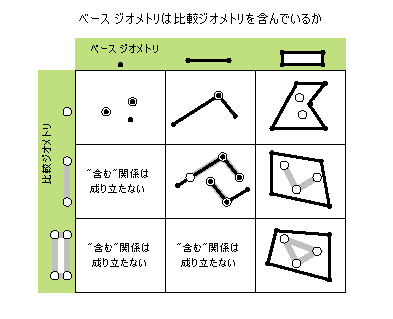crosses (second_geometry) Indicates if the two geometries intersect in a geometry of a lesser shape type. Two polylines cross if they share only points in common, at least one of which is not an endpoint. A polyline and an polygon cross if they share a polyline or a point (for vertical line) in common on the interior of the polygon which is not equivalent to the entire polyline. Only True relationships are shown in this illustration.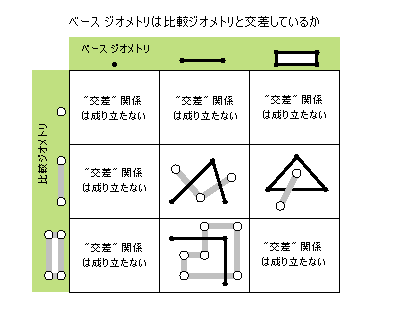disjoint (second_geometry) Indicates if the base and comparison geometries share no points in common. Two geometries intersect if disjoint returns False. Only True relationships are shown in this illustration.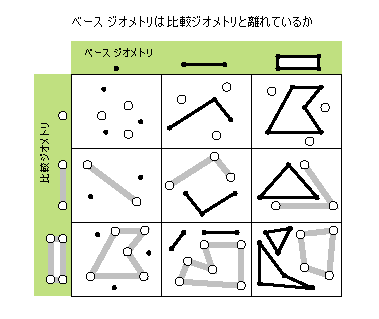equals (second_geometry) Indicates if the base and comparison geometries are of the same shape type and define the same set of points in the plane. This is a 2D comparison only; M and Z values are ignored.Only True relationships are shown in this illustration.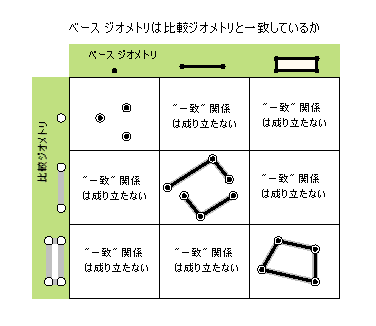overlaps (second_geometry) Indicates if the intersection of the two geometries has the same shape type as one of the input geometries and is not equivalent to either of the input geometries. Only True relationships are shown in this illustration.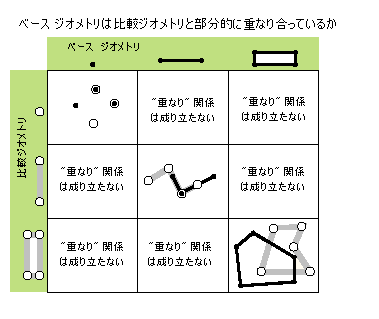projectAs (spatial_reference, {transformation_name}) Projects a geometry and optionally applies a geotransformation. To project, the geometry needs to have a spatial reference, and not have an UnknownCoordinateSystem. The new spatial reference system passed to the method defines the output coordinate system. If either spatial reference is unknown the coordinates will not be changed. The Z- and measure values are not changed by the ProjectAs method. touches (second_geometry) Indicates if the boundaries of the geometries intersect. Two geometries touch when the intersection of the geometries is not empty, but the intersection of their interiors is empty. For example, a point touches a polyline only if the point is coincident with one of the polyline end points. Only True relationships are shown in this illustration.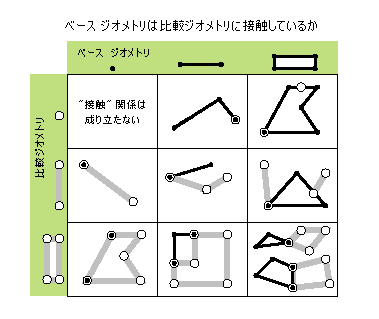within (second_geometry, {relation}) Indicates if the base geometry is within the comparison geometry. within is the opposite operator of contains. Only True relationships are shown in this illustration.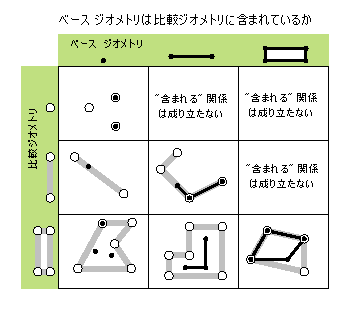The base geometry is within the comparison geometry if the base geometry is the intersection of the geometries and the intersection of their interiors is not empty. within is a Clementini operator, except in the case of an empty base geometry.

手法

contains (second_geometry, {relation})
 パラメーター 説明 データ タイプ second_geometry A second geometry. Object relation The spatial relationship type.BOUNDARY — Relationship has no restrictions for interiors or boundaries.CLEMENTINI — Interiors of geometries must intersect. Specifying CLEMENTINI is equivalent to specifying None. This is the default.PROPER — Boundaries of geometries must not intersect.(デフォルト値は次のとおりです None) String

 データ タイプ 説明 Boolean A return Boolean value of True indicates this geometry contains the second geometry.
crosses (second_geometry)
 パラメーター 説明 データ タイプ second_geometry A second geometry. Object

 データ タイプ 説明 Boolean A return Boolean value of True indicates the two geometries intersect in a geometry of a lesser shape type.
disjoint (second_geometry)
 パラメーター 説明 データ タイプ second_geometry A second geometry. Object

 データ タイプ 説明 Boolean A return Boolean value of True indicates that the two geometries share no points in common.
equals (second_geometry)
 パラメーター 説明 データ タイプ second_geometry A second geometry. Object

 データ タイプ 説明 Boolean A return Boolean value of True indicates that the two geometries are of the same shape type and define the same set of points in the plane.
overlaps (second_geometry)
 パラメーター 説明 データ タイプ second_geometry A second geometry. Object

 データ タイプ 説明 Boolean A return Boolean value of True indicates the intersection of the two geometries has the same dimension as one of the input geometries.
projectAs (spatial_reference, {transformation_name})
 パラメーター 説明 データ タイプ spatial_reference The new spatial reference. This can be a SpatialReference object or the coordinate system name. SpatialReference transformation_name The geotransformation name. String

 データ タイプ 説明 Object The projected geometry.
touches (second_geometry)
 パラメーター 説明 データ タイプ second_geometry A second geometry. Object

 データ タイプ 説明 Boolean A return Boolean value of True indicates the boundaries of the geometries intersect.
within (second_geometry, {relation})
 パラメーター 説明 データ タイプ second_geometry A second geometry. Object relation The spatial relationship type.BOUNDARY — Relationship has no restrictions for interiors or boundaries.CLEMENTINI — Interiors of geometries must intersect. Specifying CLEMENTINI is equivalent to specifying None. This is the default.PROPER — Boundaries of geometries must not intersect.(デフォルト値は次のとおりです None) String

 データ タイプ 説明 Boolean A return Boolean value of True indicates this geometry is contained within the second geometry.

コードのサンプル

Extent example

Display extent object properties for features.

import arcpy

feature_class = 'c:/Data/Florida.gdb/airports'

# Fetch each feature from the cursor and examine the extent properties
for row in arcpy.da.SearchCursor(feature_class, ['SHAPE@', 'CNTY_NAME']):
extent = row.extent
print('Extent of county {}:'.format(row))
print('XMin: {}, YMin: {}'.format(extent.XMin, extent.YMin))
print('XMax: {}, YMax: {}'.format(extent.XMax, extent.YMax))

1. 範囲 (環境設定)